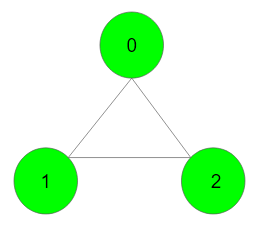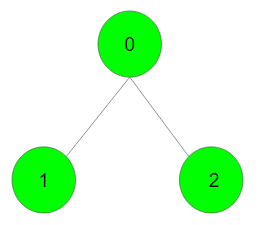XDAYS

:

HOUR

:

MINS

:

SEC

Copied to Clipboard
Euler circuit and Path
Medium Accuracy: 59.13% Submissions: 7029 Points: 4

Eulerian Path is a path in graph that visits every edge exactly once. Eulerian Circuit is an Eulerian Path which starts and ends on the same vertex. The task is to find that there exists the Euler Path or circuit or none in given undirected graph with V vertices and adjacency list adj.

Example 1:

Input:Output: 2
Explanation: The graph contains Eulerian circuit


Example 2:

Input:Output: 1
Explanation: The graph contains Eulerian path.


You don't need to read or print anything. Your task is to complete the function isEulerCircuilt() which takes number of vertices in the graph denoting as V and adjacency list of graph denoting as adj and returns 1 if graph contains Eulerian Path, 2 if graph contains Eulerian Circuit 0 otherwise.

Expected Time Complexity: O(V+E) where E is the number of edges in graph.
Expected Space Complexity: O(V)

Constraints:
1 ≤ V, E ≤ 104

### Editorial

We strongly recommend solving this problem on your own before viewing its editorial. Do you still want to view the editorial?

#### My Submissions: